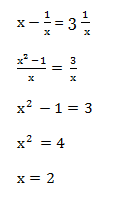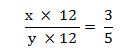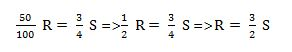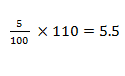# RRB ALP 2018 Practice Test Papers | Arithmetic Questions (Day-79)

Dear Aspirants, Here we have given the Important RRB ALP & Technicians Exam 2018 Practice Test Papers. Candidates those who are preparing for RRB ALP 2018 can practice these Arithmetic Questions to get more confidence to Crack RRB 2018 Examination.

[WpProQuiz 3117]

Click “Start Quiz” to attend these Questions and view Solutions

1) Arun Can type 40 pages in an hour and Vijay can type 60 pages an hour. How many pages can be typed if  they work together?

a) 48 pages

b) 24 pages

c) 30 pages

d) 12 pages

2) When a reciprocal of a number is subtracted from the number gives thrice of its reciprocal. What is the number?

a) 1

b) 2

c) 3

d) 4

3) In a Business, The profit ratio is 3 : 5 and the period of investment is 12 months, What is the possible investment amounts?

a) 8000 and 10000

b) 6000 and 10000

c) 10000 and 15000

d) 12000 and 7000

4) 50% of R = ¾ of S. What is the ratio of S: R?

a) 3: 1

b) 1: 3

c) 2: 3

d) 3: 2

5) 3454 + 9078 + 8687 + 9896 = ?

a) 22178

b) 22187

c) 31115

d) 22890

6) In a school, average age of boys is 70 and average age of total student is 150. What is the average age of total girls?

a) 80

b) 75

c) 64

d) Can’t be determined

7) The base and height of a triangle is 35 cm and 56 cm. Find the area of Triangle.

a) 1098

b) 980

c) 780

d) 560

8) A man bought bananas at the cost of Rs. 5 per dozen. If he earns 10 % profit on its cost price, he has to sell it per dozen at the price of

a) Rs. 7

b) Rs. 6

c) Rs. 5. 5

d) Rs. 8

9) A Boat can row upstream 16 km in 4 hours and downstream in 2 hours. What is the ratio of speed of Stream and speed of Boat?

a) 1: 3

b) 1: 4

c) 1: 2

d) 1: 5

10) Five laptop and Three mobile phone rate is 10,000. Find the rate of 15 mobile phone and 25 laptops.

a) 20,000

b) 40,000

c) 50,000

d) 60,000

Arun – 1/40

Vijay – 1/60

Arun + vijay = 1/40 + 1/ 60 = (3 + 2)/120 = 24 pages

Let the number is xInvestment amount * Time = Investment ratio6000 and 10000 only the option in the ratio of 3: 5. So it’s the possible investment amountS : R = 2 : 3

3454 + 9078 + 8687 + 9896 = 31115

There no data about total number of boys and girls. We can’t calculate the average.

Area of Triangle = ½ * Base * height = ½ * 35 * 56

35 * 28 = 980 cm2Upstream = 4 kmph                        Downstream = 8kmph

Speed of the boat = (8 + 4)/ 2 = 6 kmph

Speed of the stream = (8 – 4)/2 = 2

Speed of stream: Speed of Boat = 2 : 6 = 1 : 3

Laptop = x;                Mobile phone = y

5x + 3 y = 10000 (×5) => 25x+15 y

25x+15 y= 50000

RRB ALP 2018 – “All in One” Study Materials and Practice Sets